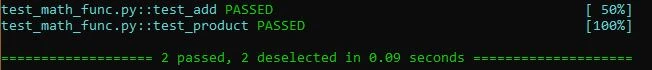60 Pages

## Unit Testing with pytestEdit

### IntroEdit

When writing test files we will want to add the 'test_' prefix to the file and the test functions in that file.

Here is a simple example script and test:

math_func.py

```def add(x, y=2):
return x + y

def product(x, y=2):
return x * y
```

test_math_func.py

```import math_func

# Define test functions.
# Expected results of the add function.
assert math_func(5) == 7

def test_product():
# Expected results of the product funciton.
assert math_func.product(5, 5) == 25
assert math_func.product(5) == 10
assert math_func.product(7) == 14
```

Then run 'test_math_func' in terminal (Anaconda Prompt if using Windows) and you should get the following:

As you can see, our tests all passed. We can use the '-v' (verbose) flag to show us a little more information about which tests have passed and which ones have failed. (I have made one of the add tests fail for this example).

it willl also give us a display of where the tests have failed in more detail.

### OptionsEdit

#### Run Specifin Tests (::)Edit

Lets say you wanted to only run one of the tests in your test file, you can do that using the following syntax:

```pytest <file>::<testName>
```

For example:

```pytest test_math_func.py::test_add
```

#### Run tests by keyword expressions (-k)Edit

Another option you can use is with '-k'. This will run tests which contain names that match the given string expression. Such as operators, file names, class names, and function names.

For example:

Say we just want to run the tests that contain the "add" keyword. We  could run:

```pytest -v -k "add"
```

And it would test the following:

Also if you write say:

```pytest -v -k "add or string"
```

it will run tests with 'add' OR string 'keywords'. For example:

You can do the same thing with 'and'.

#### Marker expression (-m)Edit

We can add markers to our test file using decorators like the following:

```import math_func
import pytest

# In this test file we have two markers, 'number' and 'string'.

@pytest.mark.number
# Define test functions.
# Expected results of the add function.
```

Then in the terminal we can run this:

```pytest -v -m number
```

and it will run all the tests that have the marker 'number'.

###Edit

#### Stopping after the first (or N) failures (-x)Edit

If we want the testing process to stop after the first (N) failures we simply run:

To stop after the first failure:

```pytest -x
```

To stop after two failures:

```pytest --maxfail=2
```

#### Modifying TracebackEdit

We can turn off the traceback all together by typing:

```pytest -v --tb=no
```

#### Skip MarkerEdit

We can skip over markers by doing the following.

Add the skip marker to our test with a given reason to print.

```@pytest.mark.skip(reason='do not run number add test')
# Define test functions.
# Expected results of the add function.
```

Then in terminal we can run

```pytest -v
```

And it will skip that given test.

You can run

```pytest -v -rsx
```

And it will print out the reason for the test skip that you passed to the skip decorator.

You can also conditionally skip tests using 'skipf' in your marker. It might look something like this:

```# Skip if python version is less than 3.3.
@pytest.mark.skipf(sys.version_info < (3, 3), reason="blah blah blah")
```

#### Show print statementEdit

Say if we add a print statement to one of our tests like the following:

```def test_add():
# Expected results of the add function.
```

We can then run

```pytest -v -s
```

and it will print the print statement(s):

#### Quiet Mode (-q)Edit

No extra information will be printed, just whether not tests were passed.

### ParametrizeEdit

This is a way of using the same function to test different types of values without having to rewrite function over and over again. So for example, instead of writing this:

```def test_add():
# Expected results of the add function.

assert result == 'Hello World'

assert result == 36
```

```@pytest.mark.parametrize('num1, num2, result',
[
(7, 3, 10),
('Hello', ' World', 'Hello World'),
(10.5, 25.5, 36)
]
)
# Expected results of the add function.
```

## TaskEdit

The Task structure is used as a data structure to pass information between the UI and API.

Here is an example of using Task:

```from collections import namedtuple

Task.__new__.defaults__ = (None, None, False, None)

def test_member_access():
Check.field functionality of namedtuple